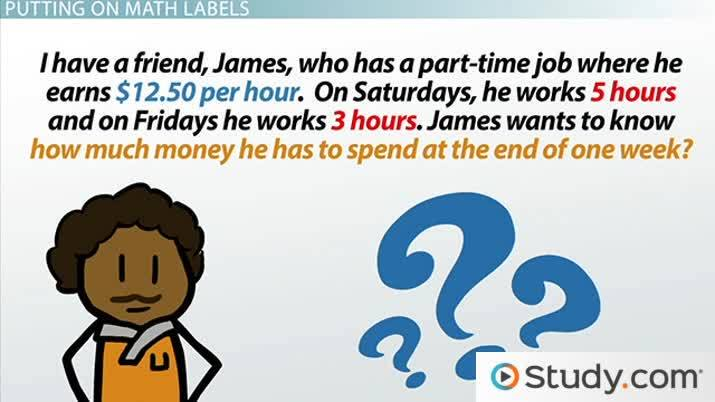Math Courses / Course / Chapter

# What is the Correct Setup to Solve Math Problems?: Writing Arithmetic Expressions

Lesson Transcript
Instructor: Yuanxin (Amy) Yang Alcocer

Amy has a master's degree in secondary education and has been teaching math for over 9 years. Amy has worked with students at all levels from those with special needs to those that are gifted.

It is important to correctly setup math problems because it makes them much easier to solve for the answer. Discover more about the correct setup, including using math labels, writing the arithmetic expression, and solving the problem.

## A Math Problem

We begin with a problem. I have a friend, James, who has a part-time job where he earns \$12.50 per hour. On Saturdays, he works 5 hours and on Fridays he works 3 hours. James wants to know how much money he has to spend at the end of one week.An error occurred trying to load this video.

Try refreshing the page, or contact customer support.

Coming up next: Understanding and Evaluating Math Formulas

### You're on a roll. Keep up the good work!

Replay
Your next lesson will play in 10 seconds
• 0:01 A Math Problem
• 0:17 Putting on Math Labels
• 1:48 Writing the Math Expression
• 3:34 Solving the Problem
• 4:11 Lesson Summary
Save Timeline
Autoplay
Autoplay
Speed Speed

## Putting on Math Labels

The first step in correctly setting up our math word problem is to label the important parts of the problem. By important, I mean the parts of the problem that we need to use to solve the problem. How do we know what these are? We begin by looking for the kind of answer the problem wants. In our problem, the problem is asking about the total amount earned at the end of a week. I go ahead and highlight the phrase that says that: how much money he has to spend at the end of one week. Next, I think of what I need to calculate that answer. Well, I need to know how much he worked and how much he makes. The problem tells me that he makes \$12.50 per hour. I can highlight this part. The problem also tells me that he works 5 hours on Saturday and 3 hours on Fridays. I go ahead and highlight this information also. Do I need anything else to solve? No, so I can ignore the other words in the problem.

Now, I can label these parts of the problem. I am going to label what I am looking for, my answer, with an x. I write this x next to the highlighted part of the problem that tells me what to solve for. I can abbreviate to 'S' for Saturday's earnings and 'F' for Friday's earnings.

## Writing the Math Expression

I am now done with labeling and now need to write my math expression that will allow me to solve the problem easily. This part requires a bit of thinking, but it's worth it!

I begin writing my math expression by putting down x = since I know that my answer equals something. I put down x for my answer part that I've labeled, and I put the equals sign down to let me know that I need to solve something to find my answer. What goes after the equals sign? Well, the problem wants to know how much James earns in a week. If James only works on Fridays and Saturdays, then the total amount of earnings for the week will be his Friday earnings plus his Saturday earnings. I've labeled those parts of the problem already, so I am going to write those labels down. I can either write 'Friday earnings' or 'F,' and I can write 'Saturday earnings' or 'S'. I put a plus sign in between these two parts to let me know that I need to add them together. So, now my math expression looks like x = F + S. But what is F and S? If James gets paid \$12.50 per hour, then the amount he earns each day depends on how many hours he works. I need to multiply his earnings by the number of hours he works. For Friday it would be \$12.50*3, and for Saturday it would be \$12.50*5. So, I can replace the 'F' with \$12.50*3 and the 'S' with \$12.50*5. I now have the math problem x = \$12.50*3 + \$12.50*5.

To unlock this lesson you must be a Study.com Member.

### Register to view this lesson

Are you a student or a teacher?

Back

### Resources created by teachers for teachers

Over 30,000 video lessons & teaching resources‐all in one place.Video lessonsQuizzes & WorksheetsClassroom IntegrationLesson Plans

I would definitely recommend Study.com to my colleagues. It’s like a teacher waved a magic wand and did the work for me. I feel like it’s a lifeline.

Jennifer B.
TeacherCreate an account to start this course today
Used by over 30 million students worldwide